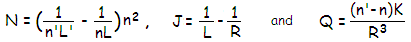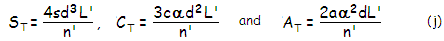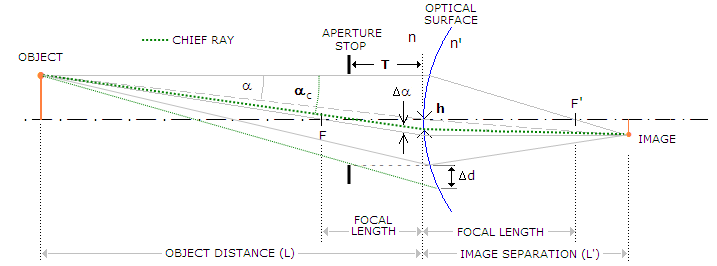telescopeѲptics.net          ▪▪▪▪                                             CONTENTS

# 3.5. OPTICAL Aberration function, Introduction

Aberration function, as the term implies, describes the size of aberration as a function of its determining factors. Since aberrations result from the interaction of light with optical surface, these determining factors belong to these three main groups:

(1) properties of light falling onto optical surface,
(2) properties of the surface, and
(3) properties of the aberration itself.

Properties of light falling onto optical surface are determined by:
- wavefront curvature or, in terms of rays, degree of convergence/divergence (i.e. object distance) , and
- its speed, dependant on the incident (object space) medium,

Properties of the surface are determined by its:
- vertex radius of curvature,
- conic,
- diameter, and
- medium type (i.e. index of reflection/refraction).

Properties of the aberration are
- its change with the radial, or radial and angular (for radially asymmetric aberrations) pupil coordinate, and
- its change with the angle of incidence.

An aberration can be expressed in various forms; longitudinal and transverse aberration forms are ray based (geometrically), while wavefront and phase deviations are OPD-related (optical path difference, also ray based, but expressing different aspects of deviation, measured in units of wave and phase deviation, respectively).

The most important aspect of aberration is the optical path difference (OPD) between the chief ray and other rays originating from the same object point at the focus location. Optical paths and OPD values are calculated using Snell's law of refraction, and/or Fermat's principle, details of which are omitted, being requiring extensive calculations.

Once the optical path lengths, i.e. specific optical path differences for sufficient number of rays are known, the form of the wavefront can also be determined. With the form of wavefront known to a sufficient accuracy, phase deviations in the image space - determining diffraction effect, i.e. change in energy distribution - can be specified as well.

A complete expression for any aberration form includes all the relevant parameters listed above; however, parameters related to the aberration properties - i.e. its change with the pupil coordinate and angle of incidence - can be, and routinely are omitted for simplicity and/or ease of calculation when multiple contributing surfaces are present (the radial pupil coordinate has to be compensated for in some form if marginal ray height varies from one surface to another but, since surface contributions are commonly calculated for each specific aberration alone, angular pupil coordinate and angle of incidence factors can be applied only to the coefficient sum).

Also, since image vs. object magnification depends on some of these same basic parameters (object and image distance, surface radius of curvature, medium), it can be used for the alternative forms of expression, particularly for mirror surface.

An aberration expression omitting both, pupil and angle of incidence factors, is called aberration coefficient. Thus, it in effect expresses the relative form of aberration for unit pupil radius and angle. The expression that includes the actual pupil parameters is the peak aberration coefficient. In this section, focus is on single-surface aberration coefficients for the three primary aberrations affecting point-image quality - spherical, coma and astigmatism, as well as generalized aberration coefficient for two or more surfaces combined; following page presents a common form of the aggregate wavefront aberration function, in terms of aberration coefficients and pupil coordinates, and its relation to the original aberration expressions formulated by von Seidel.

Stop at the surface

Wavefront aberrations at a single optical surface with the stop at the surface, either reflecting or refracting (so called general surface), for the three primary aberrations affecting point-image quality, can be expressed beginning with these three aberration coefficients:

s = -(NJ2+Q)/8            (f)

for spherical aberration, with the peak-to-valley wavefront error at paraxial focus Ws=sd4

c = 0.5NJ             (g)

for coma, with the peak-to-valley wavefront error at Gaussian image point Wc=2cαd3cosθ, for θ=0, and

a = 0.5N             (h)

for astigmatism, with the peak-to-valley wavefront error at Gaussian image point Wa=aα2d2cos2θ, for θ=0,
where(i)

n, n' being the refractive indici of the incident and refractive/reflecting media, and L, L' the object and image separation from the surface (the latter found using Gaussian approximation), respectively, R being the surface radius of curvature, and K being the surface conic. For object at infinity, L=∞ and L'=f (focal length), thus N=n/f and J=-(1/R), with Q unchanged.

The three aggregate parameters N, J and Q fully describe these three aberration coefficients. As mentioned, an aberration coefficient only includes aberration parameters related to the properties of incident light (including medium), and surface shape, while omitting those related to the pupil and angle of incidence. As such, it is meaningless as an indicator of the magnitude of aberration; it can be used as a measure of its magnitude only if associated with the pupil radius parameter d, for radially symmetrical aberrations, like spherical and defocus, and for radially asymmetric aberrations with specified pupil angle θ; for off-axis aberrations like coma and astigmatism, it also requires inclusion of the incident angle α.FIGURE 27: Main parameters of the ray geometry at a refracting optical surface with the stop at the surface: object distance L, image distance L', refractive index of the incident media n, and of the transmitting media n', surface radius of curvature R and field angle
α=α', which equals the chief ray angle. They are the basis for calculating optical path difference (OPD) vs. chief ray path, and the magnitude of aberrations. With the stop at the surface, rays from any abaxial image point are passing through the surface, center is identical to the field angle, and chief ray angle coincides with field angle.

Note that the P-V wavefront deviations are those for classical primary aberrations, at paraxial focus; d is the aperture radius, α the field angle in radians (identical to the chief ray angle for that point height) and θ the pupil angle (describes the form of rotationally asymmetrical aberrations). By setting θ to zero for coma and astigmatism, the relations give the maximum, P-V error, along the axis of aberration.

Relations for c and a imply that, with the aperture stop at the surface, coma and astigmatism are independent of the surface conic.

If aspheric coefficient b is present, it is added to the sum in the brackets in (f).

For mirror surface, one of the three basic parameters can be written in a different form, simplifying the coefficient expressions: (1/n'L')-(1/nL)=2/nR, hence N=n/R or, for mirror in air oriented to the left, N=1/R. Also, J=(1/L)-(1/R)=R(m+1)/(m-1), with m being the mirror image magnification factor. For object at infinity, m=0 and J=1/R.

Table below summarizes the three aberration coefficients for the stop at the surface and object at infinity.

 ABERRATION ABERRATION COEFFICIENT P-V WAVEFRONT ERROR at Gaussian image point General surface Mirror surface Lens surface (ng=glass index) Primary spherical s=[(2n/n'fR2)+(n'-n)K/R3]/8 s=(1+K)/4R3 s=[(2/ngfR2)+(ng-1)K/R3]/8 Ws=sd4 Primary coma c=-n/2n'fR c=1/R2 c=-1/2ngfR Wc=2cαd3 Primary astigmatism a=n/2f a=1/R a=1/2f Wa=aα2d2

TABLE 2: Aberration coefficients for the three image quality aberrations (as opposed to the image form aberrations, field curvature and distortion) and the corresponding P-V wavefront error relations

Likewise, transverse aberrations, also in the paraxial image space, are given by:

ST = -(NJ2+Q)d3L'/2n' for primary spherical aberration, as the radius of paraxial blur,
CT = 3NJd2L'α/2n' for primary coma, as the length of coma blur (tangential coma), and
AT = -NdL'α2/n' for primary astigmatism, as the diameter of the smallest blur

Transverse ray aberration can also be expressed in terms of the aberration coefficients, as:for spherical aberration, coma and astigmatism, respectively. For object at infinity, L'=f; substituting -1 for n' gives transverse aberrations for mirror surface and object at infinity as TS=-4sfd3=-2sRd3=-(1+K)d3/2R2 for spherical aberration, TC=-3fαd2/R2 for coma and TA=dα2 for astigmatism.

The corresponding longitudinal aberration is simply larger by a L'/d factor, or SL=4sd2L'2/n', and AL=2aα2L'2/n' (coma does not have the longitudinal component).

EXAMPLE: A 150mm diameter f/10 concave spherical mirror, oriented to the left, d=75mm, R=-3000mm, n=1, n'=-1 (since light reverses direction, but the medium is unchanged), for object at infinity, so L=-∞ and L'=R/2=f=-1500mm. This gives N=-1/L'=-2/R, J=-1/R2, and Q=0, resulting in s=2/8R3=9.26-12 and S=Ws=sd4=-0.000293mm, c=1.1-7 (or 1.1/107=0.00000011) and C=Wc/2=cαd3=0.047α, a=0.00067 and A=Wa=a(αd)2=1.88α2 (Ws, Wc, and Wa are peak-to valley wavefront error of spherical aberration, coma and astigmatism, respectively). In units of 0.00055mm wavelength, the mirror aberrations in the paraxial focus image space are 0.53 waves P-V of spherical aberration, 0.3 waves of coma and 0.01 wave of astigmatism, both for α=0.1� (as explained in more details with each specific aberration, the Gaussian focus image is not the best image; the P-V wavefront aberrations are reduced at their respective best, or diffraction focus location by a factor of 0.25 for spherical aberration, 0.33 for coma, and unchanged for astigmatism - however, the RMS wavefront error for astigmatism at the best focus is reduced by a factor of 1/1.5).

The corresponding transverse aberrations are TS=-0.0234mm as the radius of paraxial blur (the minus sign indicates it is oriented below the axis for marginal ray above the axis), TC=0.0049mm for tangential coma, and TA=0.00023mm for the smallest astigmatic blur diameter. Expressed in Airy disc diameters for 0.00055mm wavelength (F/745 in mm), the blur is 1.74, 0.36 and 0.017 for spherical aberration, coma and astigmatism, respectively.

For two-mirror system, the object is image formed by the secondary, thus its axial vertex separation from secondary's surface is numerically positive for the Gregorian, and negative for the Cassegrain. Refractive indici for the secondary are n=-1 and n'=1.

For refractive surface, n=1 and n'=nl, nl being the glass index of refraction. For lens, the object for the rear lens surface is the image formed by the front surface, thus distance to it with respect to rear surface is positive with a convex front surface and first surface object farther than the first surface focal point; the indici for the rear surface are n=nl and n'=1. The rest of parameters can be found using basic imaging relations.

The combined aberration for two or more surfaces is given as a sum of (wavefront) aberration coefficients at each surface, as s=s1+s2+..., c=c1+c2+... and a=a1+a2+..., assuming equal aperture radius at each surface. In practice, telescope systems regularly handle converging - occasionally also diverging - cones, resulting in unequal marginal ray height (i.e. effective aperture radius) at individual surfaces. Since the final size of aberration always depends on the size of aperture - expressed as peak aberration coefficients S=sd4, C=cαd3 and A=a(αd)2 for spherical aberration, coma and astigmatism, respectively - either representing the peak-to-valley wavefront error (for spherical aberration and astigmatism), or directly related to it (one half of the P-V wavefront error for coma) - combining aberration coefficients requires factoring in the aperture radius difference. It can be done either directly, by multiplying aberration coefficient with the appropriate radius term before combining aberration contribution from different surfaces into a system sum, or at the level of aberration coefficient, by correcting it for the radius term difference.

For instance, system coefficient for spherical aberration for a two-mirror system with the marginal ray heights D1 and D2 on the primary and secondary, respectively, is given by ss=s1+(D2/D1)4s2, with s1 and s2 being the individual aberration coefficients for the primary and secondary (the D2/D1 ratio, representing the relative aperture of the secondary mirror - specifically, its so called "minimum size" - in units of the primary aperture, is used in calculating two-mirror system aberrations as the k parameter).

For off-axis aberrations, however, an additional parameter needs to be accounted for: the stop position. It applies either to a single surface with a separated aperture stop, or to any multi-surface system, whether the aperture stop for the first surface coincides with it (when applies to the rest of the surfaces), or not.

Aberrations for displaced stop

With the stop displaced from the surface, the ray geometry changes (Fig. 151), with the chief ray passing not through the surface vertex, but through the center of the aperture stop. In terms of wavefront, it is relevant for off-axis points displaced laterally with respect to the surface, which results in a change of the aberration values.FIGURE 28: Separating aperture stop from surface changes the surface-light interaction by intercepting incoming light at a plane different from the surface plane, thus altering ray and wavefront geometry relative to the surface. The illustration shows identical surface, object and object distance as on FIG. , but this time with the stop displaced at distance T from the surface toward incoming light. As a consequence, the entering wavefront is now outlined at the stop, with its central ray - the chief ray for off axis object points (dotted green) - arriving at the surface off center (h). The new chief ray angle, αc, is larger (
Δα), and optical surface needs to be larger (Δd) in order to intercept all the light from off axis point passing through the stop. For object at infinity, there is no change in the chief ray angle due to displaced stop, only its point of intersection with a surface.

For the axial point (spherical aberration), there is no change in aberration coefficient as long as the effective aperture remains unchanged. If displaced stop changes what would be the effective aperture without it (normally, determined by the clear diameter of the first optical surface), the aberration coefficient is calculated for the effective aperture.

The additional factors for calculating aberration coefficient for off-axis image point are, then, the surface-to-stop separation T, and the new chief ray angle αc.

General form of surface aberration coefficient for the three Seidel aberrations affecting point-image quality when the stop is displaced from surface (hence with subscript d) is:

sd = -(NJ2+Q)/8                (k)
for spherical aberration, with the peak-to-valley wavefront error Ws=sd4 (unchanged)

cd = 0.5(NJY+Q)h               (l)
for coma, with the peak-to-valley wavefront error Wc=cd3cosθ, for θ=0, and

ad = -0.5(NY2+Q)h2           (m)
for astigmatism, with the peak-to-valley wavefront error Wa=ad2cos2θ for θ=0

with new parameters, in addition to N, J and Q, defined by Eq. (i), being Y=[(1/T)-(1/R)], and h=Tαc, the height of the chief ray at the surface, where T is the stop to surface separation and αc =α/[1-(T/L)] the chief ray angle (by definition, chief ray passes through the center of the aperture stop) with α being the field angle at the surface with the stop at it) and L, as before, the object distance.

It is immediately apparent from Eq. (l) and (m) that now coma and astigmatism too are dependant on the surface conic. The corresponding P-V wavefront error is obtained by substituting these coefficients for the zero-shift coefficients in the right most column of Table 2 (again, it is the error at paraxial/Gaussian focus, not best focus location). If aspheric coefficient b is present, it is added to the sum in the brackets for all three, spherical aberration, coma and astigmatism (not only for spherical aberration, as with the stop at the surface).

Obviously, it is the stop-to-surface separation T (according to sign convention positive for stop to the left of surface, negative when at the right) that creates the difference vs. stop at the surface geometry. When T=0, Y=1, Q=0, αc=α, and the stop-shift relations reduce to those with the stop at the surface. When the stop precedes the first surface, T is simply the stop-to-surface separation; for the following surface, the stop is at the location of the image of aperture stop formed by the preceding surface, hence T is the separation between this image (usually virtual) and the surface, and so on. Similarly, when the stop is at the first surface, like in two-mirror systems, aperture stop for the secondary is the primary. In a three-mirror system, aperture stop for the tertiary is the image of primary formed by the secondary.

So, in general, stop separation, based on Eq. (1) is given by T=n'IPR/[(n'-n)IP+nR] for general surface, with n', IP, R and n being the refractive index of incident medium, separation to the image formed by preceding surface, surface radius of curvature and refractive index of transmitting medium, respectively. For mirror surface, n'=-n and, for surface in air oriented to left, n=1, with T=-IPR/(R-2IP).

Aberration coefficient are the first level of aberration function. They only describe a single aberration, and can be used to calculate wavefront and phase deviation resulting from it. More often, telescope systems generate combined aberrations, which require more complex aberration function to describe. Follows detailed description of the general form of aberration function for the five classical, or Seidel aberrations.

3.4. Terms and conventions   ▐    3.5.1. Seidel aberrations